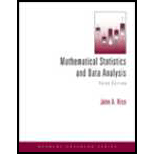# Prove that P ( u &lt; X ≤ v ) = F ( v ) − F ( u ) for any u and v in the cases that X is a discrete random variable.### Mathematical Statistics and Data A...

3rd Edition
John A. Rice
Publisher: Cengage Learning
ISBN: 9781111793715### Mathematical Statistics and Data A...

3rd Edition
John A. Rice
Publisher: Cengage Learning
ISBN: 9781111793715

#### Solutions

Chapter 2.5, Problem 5P

a.

To determine

## Prove that P(u<X≤v)=F(v)−F(u) for any u and v in the cases that X is a discrete random variable.

b.

To determine

### Want to see the full answer?

Check out a sample textbook solution.

### Want to see this answer and more?

Experts are waiting 24/7 to provide step-by-step solutions in as fast as 30 minutes!*

*Response times may vary by subject and question complexity. Median response time is 34 minutes for paid subscribers and may be longer for promotional offers.